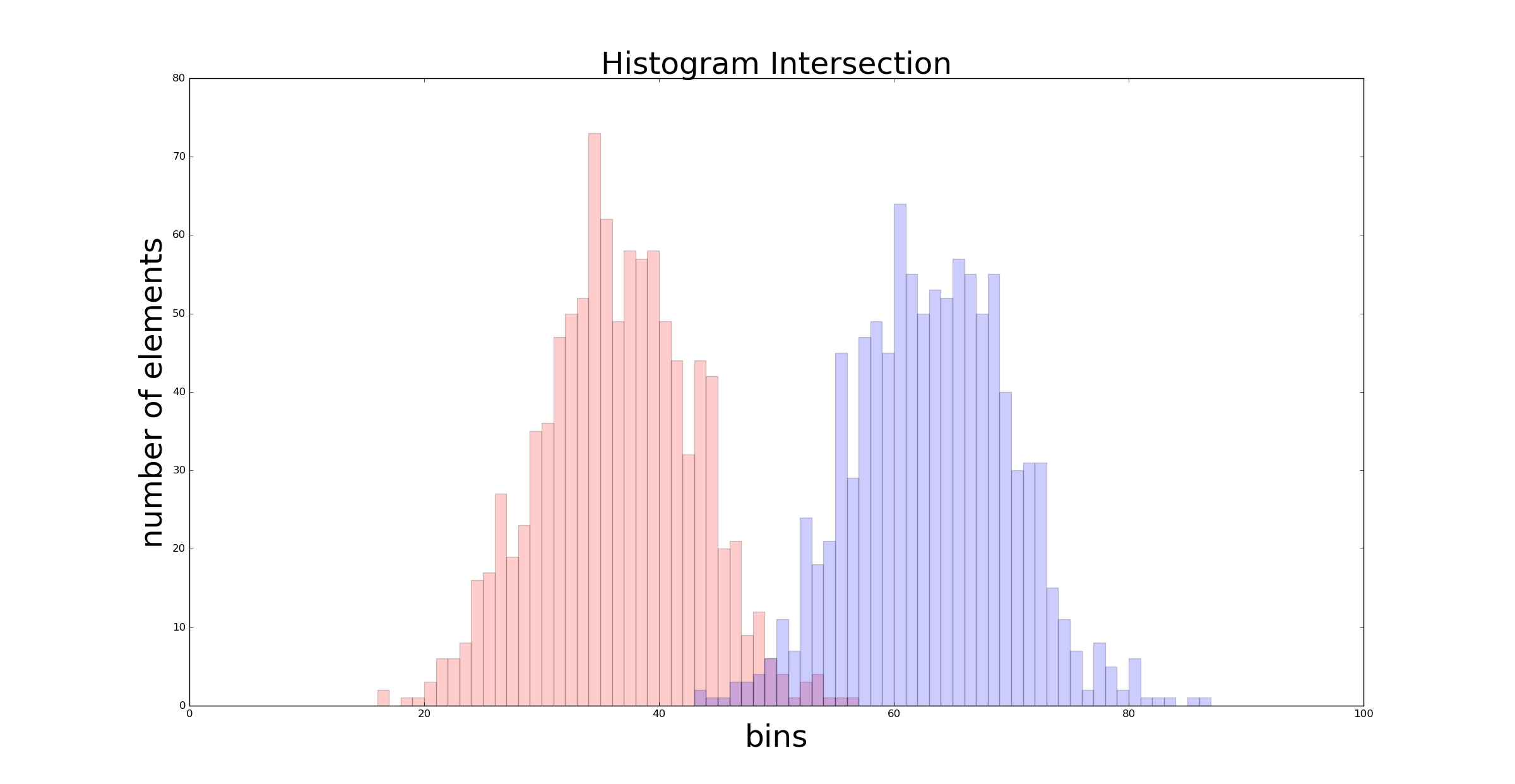How to find the threshold between two histograms at fixed false positive rate?

I have two histograms with equal bin size that lie in the same general range. You can imagine them looking like this example I've borrowed from https://mpatacchiola.github.io/blog/2016/11/12/the-simplest-classifier-histogram-intersection.html:Let's say, the blue-ish histogram corresponds to true examples, while the red-ish histogram corresponds to false examples. I want to find a threshold that can be used to predict true examples or false examples in the future. If you want, this is a machine learning problem where I want to find a binary classifier for one-dimensional data.

Obviously, a naive and straight-forward choice would be to find the local minimum in the middle of the two histograms and cut right through it. How can this be solved efficiently? My first idea would be to fit two Gaussians and intersect them.

The whole thing gets more interesting if you additionally seek to fix the false positive rate (corresponding to the red-ish bins located to the right of the threshold). How can the FPR be put into the equation?

• Note that for question 1, if you think the data is a gaussian mixture you still have to fit the mixing fraction as well as the individual gaussians (i.e. your histograms are un-normalized counts, and their totals need not be equal). For question 2, you would use the CDFs (either cumsum the histograms, or take the gaussian CDFs + their mixing fractons). – GeoMatt22 Apr 25 '17 at 21:31
• If you think both groups are Gaussian, this becomes quadratic discriminant analysis; if you think their variance / covariance matrix (in higher dimensions) is the same, then it's linear discriminant analysis. Many posts on site discuss these and related techniques. – Glen_b Apr 25 '17 at 22:37

It's actually more of a problem in Statistics.

A little bit of theory:

Consider the following formulation using hypothesis testing, which you're hopefully familiar with.

In the general setting, we observe examples $x_{1},...,x_{m}\sim f\left(x\right)$ and we have two "candidates" for the density function $f$ which the examples came from: $f_1$ and $f_2$. In the language of hypothesis testing, we have $H_{1}$ and $H_{2}$ and we want to distinguish between them.

For a simple case, which suits your scenario better, consider the case of a single example (the case for a general $n$ is very similar but it's a little bit different than what you described since it assumes all examples come from either $f_1$ or $f_2$).

Given an example $x$, we would like to choose the distribution that is most probable:

$prediction = argmax_{i=1,2}\mathbb{P}\left(H_{i}\mid x\right)$

Equivalently, we can choose $H_1$ $\iff$ $\frac{\mathbb{P}\left(H_{1}\mid x\right)}{\mathbb{P}\left(H_{2}\mid x\right)} > 1$.

Using Bayes theorem to simplify the above expression,

$\frac{\mathbb{P}\left(H_{1}\mid x\right)}{\mathbb{P}\left(H_{2}\mid x\right)} = \frac{f_{1}\left(x\right)}{f_{2}\left(x\right)} \cdot \frac{\mathbb{P}\left(H_{1}\right)}{\mathbb{P}\left(H_{2}\right)}$

Taking logarithm of both sides gives a nice decomposition (this step is not mandatory, but usually done for further analysis of the general case):

$\log\frac{\mathbb{P}\left(H_{1}\mid x\right)}{\mathbb{P}\left(H_{2}\mid x\right)}=\log \frac{f_{1}\left(x\right)}{f_{2}\left(x\right)} + \log \frac{\mathbb{P}\left(H_{1}\right)}{\mathbb{P}\left(H_{2}\right)}$

$\log \frac{\mathbb{P}\left(H_{1}\right)}{\mathbb{P}\left(H_{2}\right)}$ is known as the log likelihood ratio; it's fixed and signifies the initial inclination (without seeing any data) to choosing $H_1$; $\log \frac{f_{1}\left(x\right)}{f_{2}\left(x\right)}$ can therefore be seen as the additional information that example $x$ gave you regarding choosing $H_1$.

(In the general case, we can go on to show that - as one would anticipate - when you see more examples, the probability of choosing the correct density goes to 1.)

Practical Usage:

1. Use the information you have (histogram, etc) to fit a density estimator for your two candidate functions. This can be done in two lines of code in Python, R, etc.

2. Predict: $\hat y = argmax_{i=1,2}\hat f_{i}(x)$

• Thank you for this detailed elaboration, which was straight-forward and quite understandable. However, I have to admit that I am still not able to follow Step 2 of the suggested practical usage. Wouldn't that mean that y is located where the maximum of both distributions appears? This would directly contradict my naive understanding that we would like to find the threshold somewhere in the gap between both distributions... – Michael Apr 28 '17 at 9:05